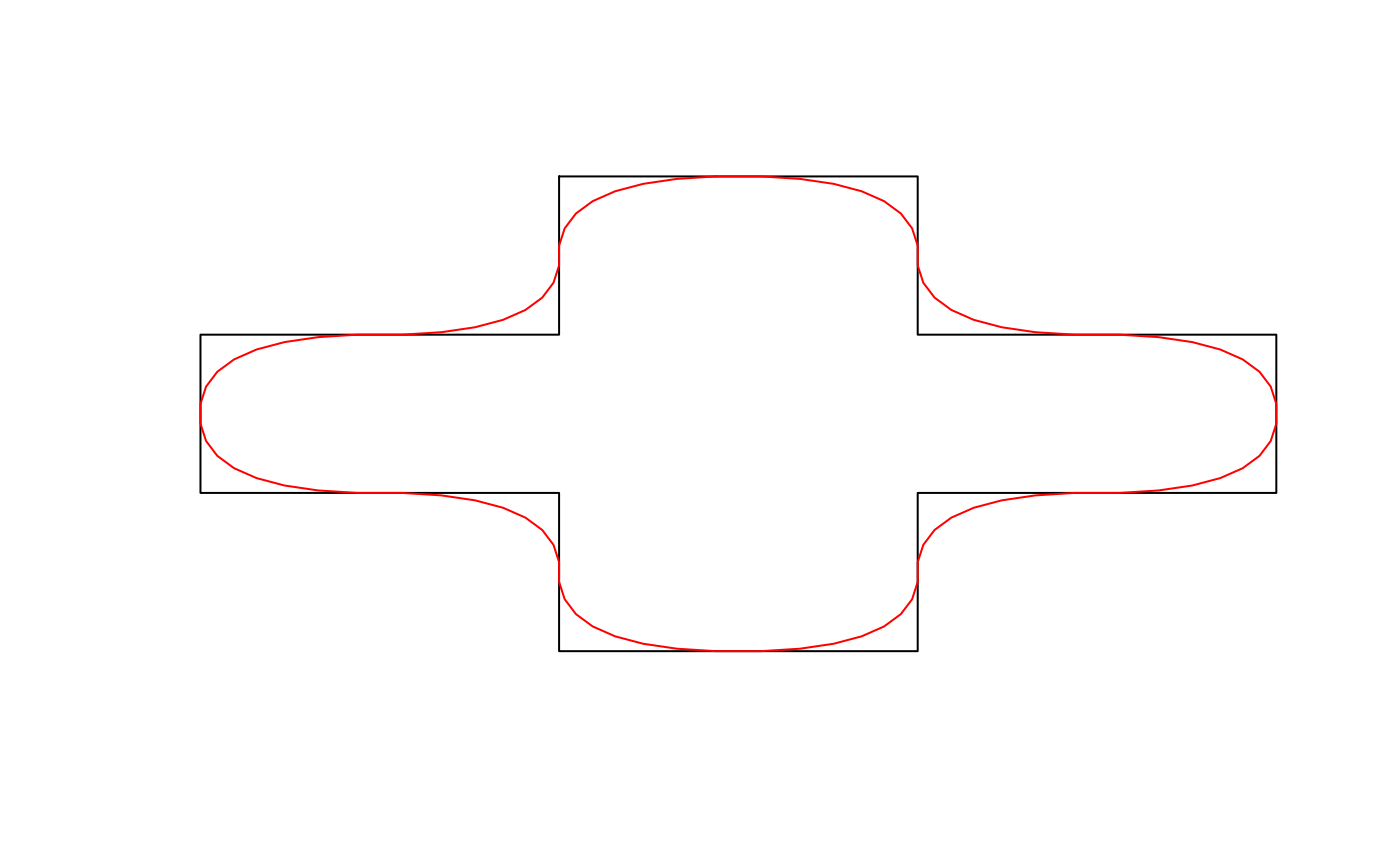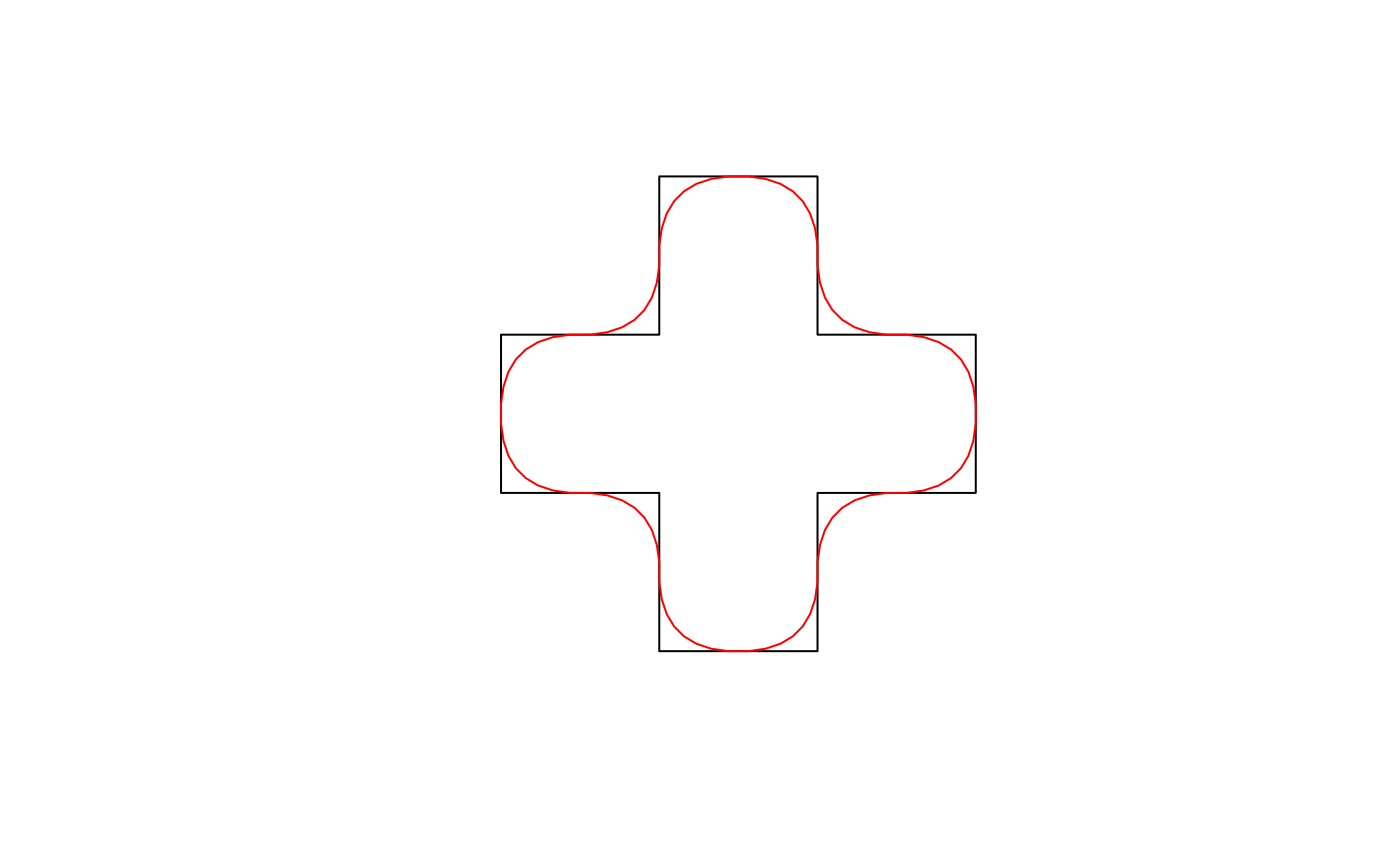Chaikin's corner cutting algorithm smooths a curve by iteratively replacing every point by two new points: one 1/4 of the way to the next point and one 1/4 of the way to the previous point.

smooth_chaikin(x, wrap = FALSE, refinements = 3L)

## Arguments

x numeric matrix; 2-column matrix of coordinates. logical; whether the coordinates should be wrapped at the ends, as for polygons and closed lines, to ensure a smooth edge. integer; number of corner cutting iterations to apply.

## Value

A matrix with the coordinates of the smoothed curve.

## Details

This function works on matrices of points and is generally not called directly. Instead, use smooth() with method = "chaikin" to apply this smoothing algorithm to spatial features.

The original reference for Chaikin's corner cutting algorithm is:

• Chaikin, G. An algorithm for high speed curve generation. Computer Graphics and Image Processing 3 (1974), 346–349

This implementation was inspired by the following StackOverflow answer:

smooth()

## Examples

# smooth_chaikin works on matrices of coordinates
# use the matrix of coordinates defining a polygon as an example
m <- jagged_polygons$geometry[][] m_smooth <- smooth_chaikin(m, wrap = TRUE) class(m) #>  "matrix" "array" class(m_smooth) #>  "matrix" "array" plot(m, type = "l", axes = FALSE, xlab = NA, ylab = NA) lines(m_smooth, col = "red")# smooth is a wrapper for smooth_chaikin that works on spatial features library(sf) p <- jagged_polygons$geometry[]
p_smooth <- smooth(p, method = "chaikin")
class(p)
#>  "XY"      "POLYGON" "sfg"    class(p_smooth)
#>  "XY"      "POLYGON" "sfg"    plot(p)
plot(p_smooth, border = "red", add = TRUE)